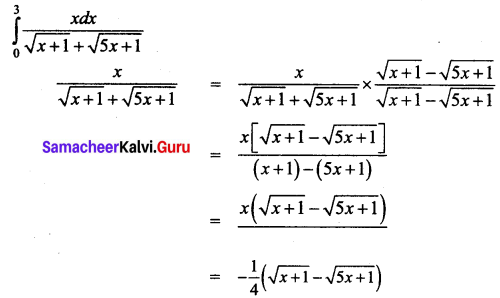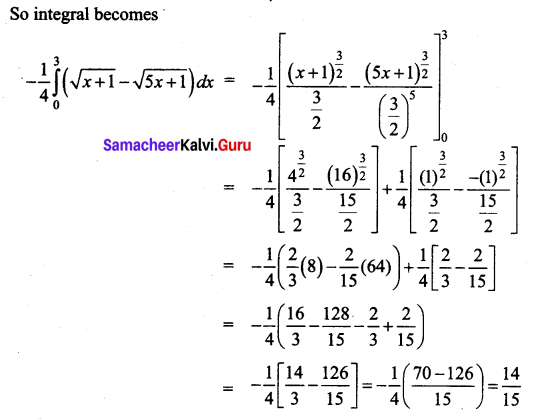Students can download 12th Business Maths Chapter 2 Integral Calculus I Miscellaneous Problems and Answers, Samacheer Kalvi 12th Business Maths Book Solutions Guide Pdf helps you to revise the complete Tamilnadu State Board New Syllabus and score more marks in your examinations.

## Tamilnadu Samacheer Kalvi 12th Business Maths Solutions Chapter 2 Integral Calculus I Miscellaneous Problems

Evaluate the following integrals:

Question 1.
$$\int \frac{1}{\sqrt{x+2}-\sqrt{x+3}} d x$$
Solution: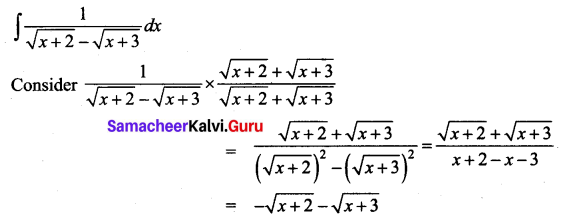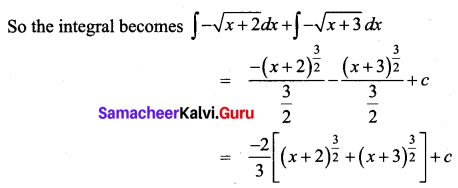Question 2.
$$\int \frac{d x}{2-3 x-2 x^{2}}$$
Solution: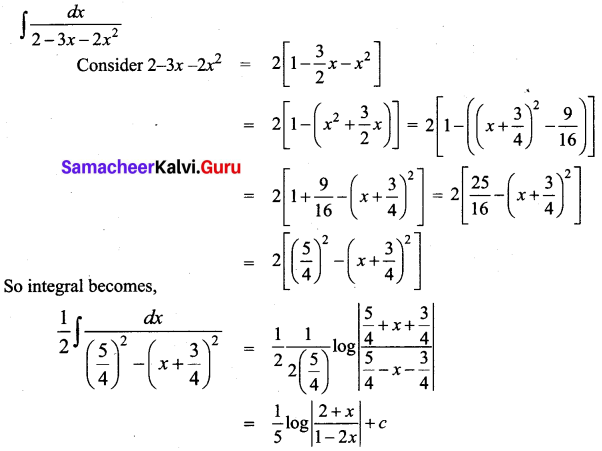Question 3.
$$\int \frac{d x}{e^{x}+6+5 e^{-x}}$$
Solution: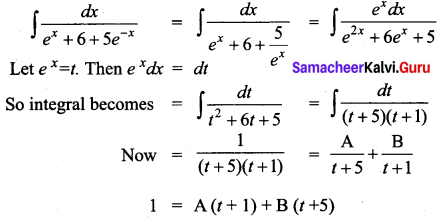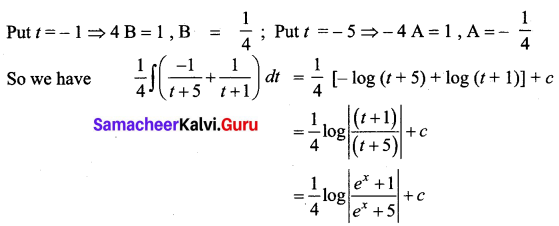Question 4.
$$\int \sqrt{2 x^{2}-3} d x$$
Solution: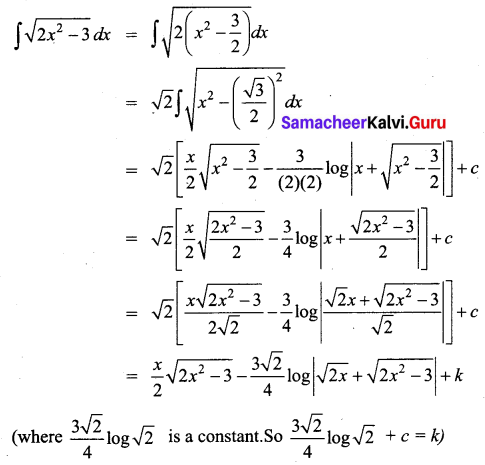Question 5.
$$\int \sqrt{9 x^{2}+12 x+3} d x$$
Solution: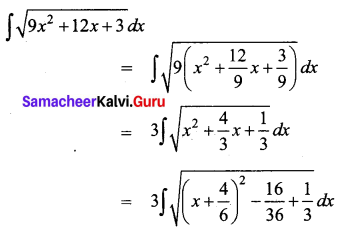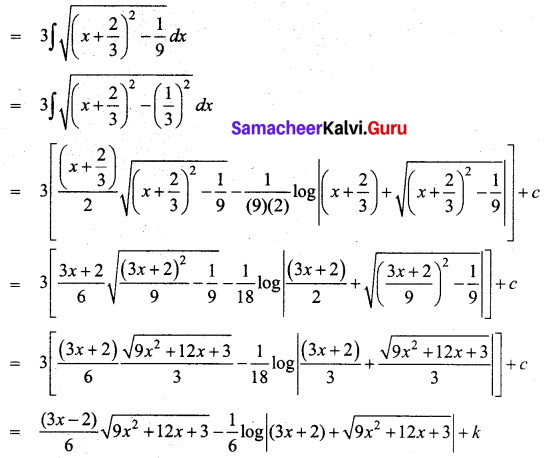Note: The constant $$\frac{1}{6}$$ log 3 can be merged with the constant ‘c’.

Question 6.
∫(x + 1)2 log x dx
Solution:
∫(x + 1)2 log x dx
We use integration by parts method.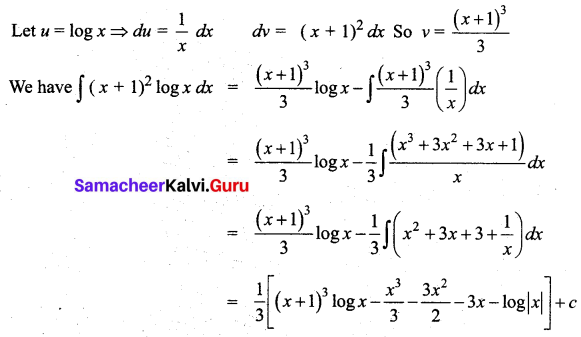Question 7.
$$\int \log (x-\sqrt{x^{2}}-1) d x$$
Solution: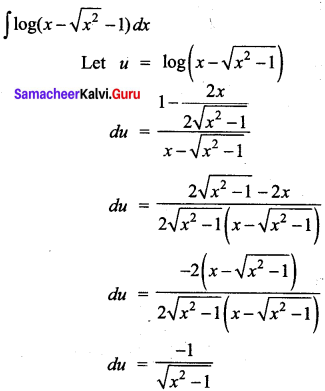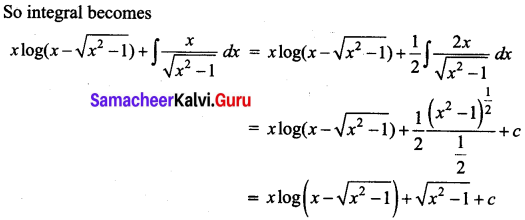Question 8.
$$\int_{0}^{1} \sqrt{x(x-1)} d x$$
Solution: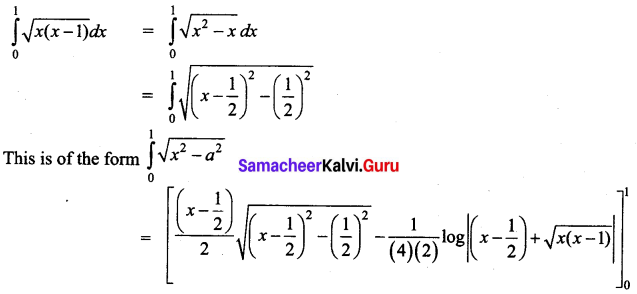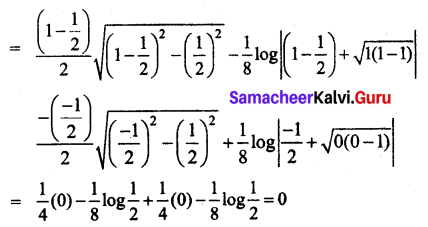Question 9.
$$\int_{-1}^{1} x^{2} e^{-2 x} d x$$
Solution: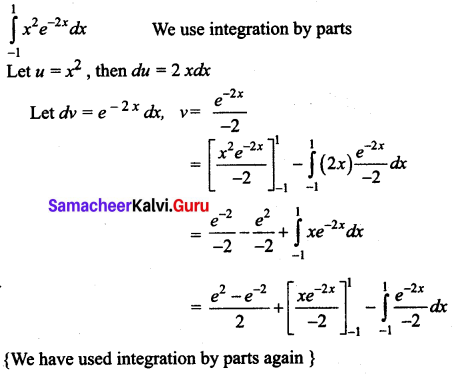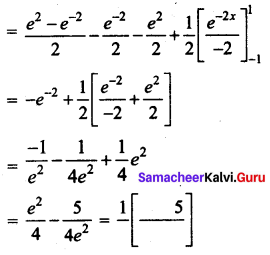Question 10.
$$\int_{0}^{3} \frac{x d x}{\sqrt{x+1}+\sqrt{5 x+1}}$$
Solution: